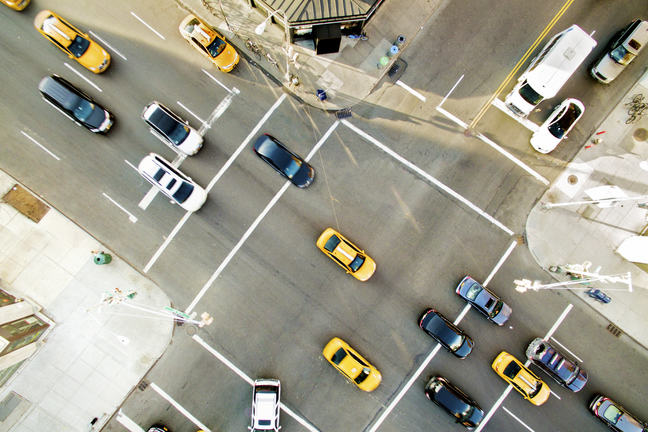## Want to keep learning?

This content is taken from the Partnership for Advanced Computing in Europe (PRACE), The University of Edinburgh & SURFsara's online course, Supercomputing. Join the course to learn more.
3.3

## Partnership for Advanced Computing in Europe (PRACE)# Traffic Simulation

Now we are going to consider how to parallelise a more interesting example than just adding up a list of numbers. First, we will describe how the example works and get familiar with it.

We are going to look at a very simple way of trying to simulate the way that traffic flows. This better illustrates how parallel computing is used in practice compared to the previous salaries example:

• We process the same data over and over again rather than just reading it once;

• The way the model works (where the behaviour of each car depends on the road conditions immediately in front and behind) is a surprisingly good analogy for much more complicated computations such as weather modelling which we will encounter later on.

### The traffic model

In this example we only talk about a straight, one-way road without any intersections. We think of the road as being divided into a number of sections or cells, each of which either contains a car (is occupied) or is empty (unoccupied). The way a car behaves is very simple: if the cell in front is occupied then it cannot move and remains where it is; if the cell in front is unoccupied then it moves forward. A complete sweep of the road, where we consider every car once, is called an iteration; each iteration, a car can only move once. Having updated the entire road, we proceed to the next iteration and check again which cars can move and which cars stand still.

If you want, you can think of each cell representing about 5 metres of road and each iteration representing around half a second of elapsed time.

Despite being such a simple simulation, it represents some of the basic features of real traffic flow quite well. We will talk you through some examples in the next few videos.

The simplest way to characterise different simulations is the density of cars, that is the number of cars divided by the number of cells. If all cells are occupied then the density is 1.0 (i.e. 100%), and if half of them are occupied then the density is 0.5 (50%).

Perhaps the simplest quantity to measure is the average speed of the cars after each iteration. If we call the top speed of a car 1.0 (i.e. it moves one cell in an iteration) then the average speed of the cars at each iteration is the number of cars that move divided by the total number of cars. For example, if we have 10 cars and all of them move then the average speed is 1.0. If only four of them move then the average speed is 0.4. If none of them move (total gridlock) then the average speed is zero.

Why do you think this problem is a good analogy for other, more complex simulations? If the traffic flow problem was to be parallelised, how do you think it could be decomposed into parallel tasks? Ask other Learners if you’re not sure!